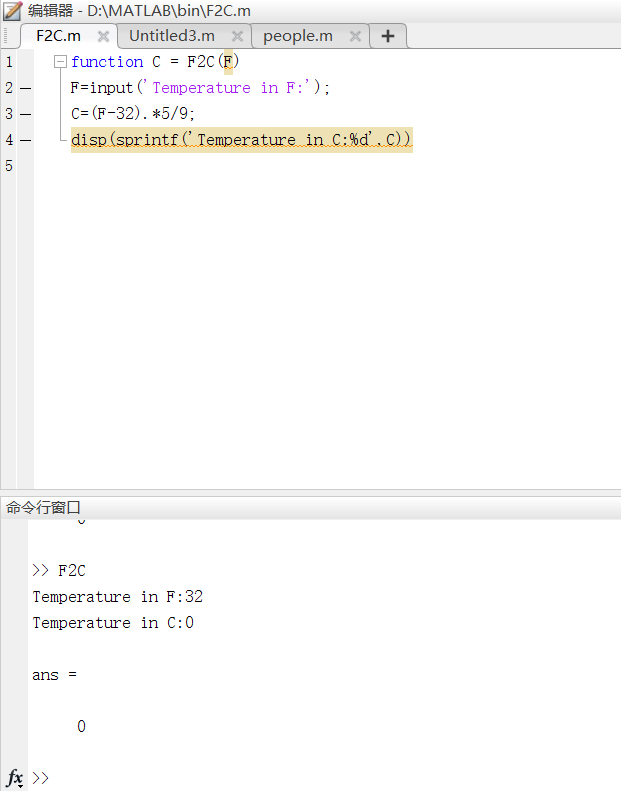• ## matlab中ans的含义

千次阅读 2019-08-12 23:28:05
当您在未指定输出参数的情况下运行返回输出MATLAB®代码时，MATLAB 会创建ans变量并将输出存储在该变量中。建议不要在脚本或函数中更改或使用ans的值，因为该值可能会经常变化。 ans特定于当前工作区。基础工作...
ns

最近计算的答案

语法

ans

说明

示例

当您在未指定输出参数的情况下运行返回输出的 MATLAB® 代码时，MATLAB 会创建 ans 变量并将输出存储在该变量中。建议不要在脚本或函数中更改或使用 ans 的值，因为该值可能会经常变化。

ans 特定于当前工作区。基础工作区和每个函数工作区可以有自己的 ans 实例。有关详细信息，请参阅基础和函数工作区。

示例

简单计算的结果

Try This Example

在命令行窗口中执行简单计算，而不将结果赋给变量。MATLAB 将结果存储在 ans 变量中。

2 + 2

ans = 4

在命令行窗口中执行简单计算，并将结果赋给变量 result。

result = 4 + 4

result = 8

显示 result 的值，然后显示 ans 的值。MATLAB 显示 result 的值而不返回输出。因此，ans 的值保持不变。

result

result = 8

ans

ans = 4

调用返回输出的函数

Try This Example

假设您有函数 testFunc，它返回输出但不指定输出变量。

function a = testFunc
a = 75;
end

调用 testFunc。MATLAB 将返回的结果存储在 ans 中。

testFunc

ans = 75

展开全文• ans=1matlab里面的ans是一个系统量，当m文件中出现非赋值性的计算时，matlab会把结果自动存入ans。如果m文件中每一个计算都是赋值的，即所计算的结果都是保存在自己设定的变量中的，那么ans一直没有得到使用，就会...
ans=1matlab里面的ans是一个系统量，当m文件中出现非赋值性的计算时，matlab会把结果自动存入ans。如果m文件中每一个计算都是赋值的，即所计算的结果都是保存在自己设定的变量中的，那么ans一直没有得到使用，就会一直是1。-----------------------------------------------解决方法：if语句引导的循环后，没加分号；导致其一直输出。加上分号即可！------------------------------------------------------------分号是用来抑制输出的~if后可以加分号~for循环后也可以加分号~---------------------------------------------------------------if语句：if.....end结构if....else...end 结构if..elseif...else...end 结构-------------------------------------------------------------for循环语句：for i=1：0.1：50；for 循环变量=表达式1:表达式2:表达式3循环体语句 ......end其中,表达式1的值为循环变量的初值，表达式2的值为步长，表达式3的值为循环变量的终值。步长为1时，表达式2可以省略。-----------------for语句更一般的格式为：for 循环变量=矩阵表达式循环体语句end执行过程是依次将矩阵的各列元素赋给循环变量，然后执行循环体语句，直至各列元素处理完毕。
展开全文• matlab里面的ans是一个系统量，当m文件中出现非赋值性的计算时，matlab会把结果自动存入ans。 如果m文件中每一个计算都是赋值的，即所计算的结果都是保存在自己设定的变量中的，那么ans一直没有得到使用，就会一直...
ans=
1
matlab里面的ans是一个系统量，当m文件中出现非赋值性的计算时，matlab会把结果自动存入ans。
如果m文件中每一个计算都是赋值的，即所计算的结果都是保存在自己设定的变量中的，那么ans一直没有得到使用，就会一直是1。

-----------------------------------------------
解决方法：
if语句引导的循环后，没加分号；导致其一直输出。加上分号即可！

------------------------------------------------------------
分号是用来抑制输出的~
if后可以加分号~for循环后也可以加分号~
---------------------------------------------------------------
if语句：
if.....end结构
if....else...end 结构
if..elseif...else...end 结构
-------------------------------------------------------------
for循环语句：
for i=1：0.1：50；
for 循环变量=表达式1:表达式2:表达式3
循环体语句 ......
end
其中,表达式1的值为循环变量的初值，表达式2的值为步长，表达式3的值为循环变量的终值。步长为1时，表达式2可以省略。
-----------------
for语句更一般的格式为：
for 循环变量=矩阵表达式
循环体语句
end
执行过程是依次将矩阵的各列元素赋给循环变量，然后执行循环体语句，直至各列元素处理完毕。
转载于:https://www.cnblogs.com/wxl845235800/p/6682574.html
展开全文• function C = F2C(F) F=input('Temperature in F:'); C=(F-32).*5/9;...程序如上，输出结果总是带有一个ans 在第一行后加；也还是带有ans 结果如下 >> F2C Temperature in F:32 Temperature in C:0 an...
function C = F2C(F)
F=input('Temperature in F:');
C=(F-32).*5/9;
disp(sprintf('Temperature in C:%d',C))

程序如上，输出结果总是带有一个ans
在第一行后加；也还是带有ans
结果如下
>> F2C
Temperature in F:32
Temperature in C:0

ans =

0

图片如下，我是Matlab新手，请大神多多指导~~展开全文• 用function写了一个函数，希望能够输出两个不同的数组，如[a,b]=fun(x),其中a，b为两个不同的数组，但是貌似b会将a的结果覆盖，得到的ans只有一个数组。程序如下： function [thta,y]=Sa(N) if N>=10000; ...开发语言
• 介绍两种方式，快速将Matlab里的表达式输出到MathType里公式编辑器里。 1. 利用符号函数和latex函数 syms x f = taylor(exp(x)) latex(f) f = x^5/120 + x^4/24 + x^3/6 + x^2/2 + x + 1 ans = \frac{x^5}{120} +...
• ans = 半波电场强度：6.900000e+01 dertaph = (100*pi)/((4722366482869645213696*pi)/5982747934487665038974042180775 + 11258999068427/2874052110483450880) ans = 相位延迟：1.000000e+02相位延迟：1...
• matlab计算结果出现如上图所示状况时，使用下列命令解决 format long g; %输出结果为小数 vpa(ans,5); %五位有效数字
• 原文地址：在MATLAB中得到系统当前日期、时间也是经常用到的内容，由以下函数实现。作者：changwanghao ...在MATLAB中得到系统当前日期、时间也是经常用到的内容，由以下函数实现。...ans =
• 运行软件：MATLAB R2019b 内置常量 >> eps // 计算机的最小数 ans = 2.2204e-16 >> pi // 圆周率 ans = 3.1416 >> i // sprt(-1) ans = 0.0000 + 1.0000i >> j // sprt(-1) ...数学建模 矩阵
• 输出ans= 3 4 2、s=size（A）%返回一个行向量s，s的第一个元素是矩阵的行数，第二个元素是矩阵的列数 输出：s= 3 4 3、[r,c]=size（A）%将矩阵A的行数返回到第一个输出变量r，将矩阵的列数返回到第二个输出...
• end 的更多用法。 >> x = [1, 2, 3, 4, 5]; >> x(end:-1:end-size(x, 2)+1) ans = 5 4 3 2 1
• 基础知识 特殊表达式 ans表示最近的值所存储的地方，eps表示浮点精度，pi为π，Inf... 要抑制和隐藏表达式的MATLAB输出，在表达式后添加分号。 符号 含义 + 相加; 加法运算符。 - 相减; 减法运算符。 ...学习笔记 基础知识
• matlab调用函数 调用max函数： A=[1 2 3]; max(A) % ans = 3 A=[1 6 3]; B=[1 2 5 ]; max(A,B) %ans = 1 6 5 通过将函数赋值给变量，返回该函数的输出 maxA=max(A) %maxA =6 如果存在多个输出参数，请将其...
• 前言 这学期学的东西都需要用到Matlab，只能强行学一波了。 基本使用方法 Matlab不仅仅功能强大，而且非常容易应用于数值运算。...函数的输出参数的个数 pi 圆周率 varagin 可变的函数输入参数的个数 ...
• 意义 ans 计算结果的变量名 computer 确定运行的计算机 eps 浮点相对精度 Inf 无穷大 I 虚数单位 inputname 输入参数名 NaN 非数 nargin 输入参数个数 nargout 输出参数的数目 pi 圆周率 ...
• 注释 使用%进行注释，选中对应区域，按Ctrl...在matlab输出的结果如果没有变量表示，默认使用ans表示： 例如： 4 ans = 4 x = 4 x = 4 在matlab中如果想要输入π，可以直接输入pi 例如： pi ans = 3.
• ans 描述 当你没有为输出指定变量的时候, MatLab会自动创建ans, 并且保存该输出 例子: 2+2 等价于 ans = 2+2 clc 描述 "Clear Command Window", 清空命令行窗口 diary 语法:...
• TIPS：可将代码复制到m文件中，一行一行运行...非常强大遇到问题首选它)MATLAB数据类型元胞数组逻辑和流程控制MATLAB的数据流控制并行处理数据可视化01常用控制命令ans %最新计算默认变量cd %设置当前工作目录clf %...
• matlab常用函数- - 1、特殊变量与常数 ans 计算结果的变量名 computer 确定运行的计算机 eps 浮点相对精度 Inf 无穷大 I 虚数单位 inputname 输入参数名 NaN 非数 nargin 输入参数个数 nargout 输出参数的数目 pi ...
• 方阵的行列式 det(A)：求方阵A所对应的行列式的值。 矩阵的秩 rank(A)：求矩阵A的秩。 >> A=[3,2,1;3,1,5;3,2,3] A = 3 2 1 3 1 5 3 2 3 >...ans = -6 >> rank(A) ans = 3 ...
• ## matlab中uint16(uint8)函数的用法

万次阅读 多人点赞 2018-10-31 15:32:56
matlab中uint16函数的用法；...0 输出ans=0; if 0&lt;=a&lt;=65535 输出ans=a; if a&gt;65535 输出ans=65535; 当输入为一个矩阵a时：对于矩阵中的每一个元素进行上述标量操作； if a=[-1 2 ...uint16 uint8
• Matlab 基础教程 教程目录Matlab 基础教程1.零碎知识2.运算符3.数据类型3.1数组3.1.1数组创建3.1.2数组索引3.1.3数组运算3.1.4数组操作3.2复数4.程序设计4.1脚本4.2实时脚本4.3程序控制...2.ans代表最近一个输出结果 3.
• 答：当在未指定输出参数的情况下，运行返回输出MATLAB 代码时，MATLAB 会创建ans变量并将输出存储在该变量中。 3、clear：在工作空间消除一个变量。clear all：在工作空间消除所有变量。 4、matlab中一个非常...
• TIPS：可将代码复制到m文件中，一行一行运行...非常强大遇到问题首选它)MATLAB数据类型元胞数组逻辑和流程控制MATLAB的数据流控制并行处理数据可视化01常用控制命令ans %最新计算默认变量cd %设置当前工作目录clf %...
• syms a b c latex(a/b) ans = '\frac{a}{b}' latex(sqrt©/a+b) ans = 'b+\frac{\sqrt{c}}{a}'
• 1、特殊变量与常数ans 计算结果的变量名 computer 确定运行的计算机eps 浮点相对精度 Inf 无穷大i 虚数单位 inputname 输入参数名NaN 非数 nargin 输入参数个数nargout 输出参数的数目 pi 圆周率nargoutchk 有效的...
• 如果表达式在未给定输出变量时，系统自动生成ans变量并将结果赋予ans。eps浮点相对精度，是计算机对于计算的容限。realmax计算机可以表示的最大浮点数realmin计算机可以表示的最小浮点数pi圆周率i,j虚...
• ans 最近计算的答案 clc 清空命令行窗口 diary 将命令行窗口文本记录到日志文件中 format 设置命令行窗口输出显示格式 home 发送光标复位 iskeyword 确定输入是否为 MATLAB 关键字 more 控制命令行......

# matlab输出ansmatlab 订阅